Our prices are denominated in USD.

We ship internationally!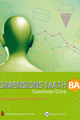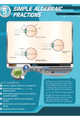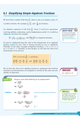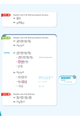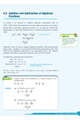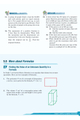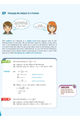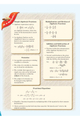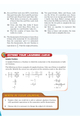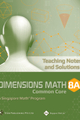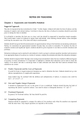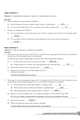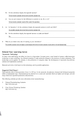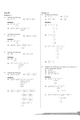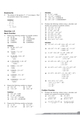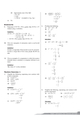# Dimensions Mathematics Common Core Textbook 8A

\$19.25

The Dimensions Mathematics Common Core Textbooks emphasize the empowerment of students to learn math independently and effectively, a variety of approaches are used to help students master integrated pre-algebra, algebra, geometry, trigonometry, and advanced math topics. This series follows the Singapore Mathematics Framework as well as the topics in the Common Core State Standards

Key Features:

• Worked examples followed by a "Try It!" question to ensure understanding.

• Lesson exercises that include the following exercise categories:
• Basic Practice (simple questions involving a direct application of the concepts);
• Further Practice (more challenging questions);
• Maths@Work (questions that apply mathematical concepts to real-life situations);
• Brainworks (questions involving higher order thinking or an open-ended approach to problems).

• Review exercise, an "Extend Your Learning Curve" activity, and "problem solving corner" exercises are also integrated throughout.

The answer key at the back of the book provides answers to the Try It! and the problems in the exercises for the Basic Practice, Further Practice, and Maths@Work questions. It does not include answers to the class activities, Brainworks questions, or the Extend Your Learning Curve activities; the answers can be found in Teacher's Notes & Solutions.

Teaching Notes and Solutions is a succinct and useful tool for educators. It contains teaching notes for each chapter, and fully worked solutions for all problems in the textbook. .

Note: Soft cover

Chapter 1: Exponents and Scientific Notation

Chapter 2: Linear Equations in Two Variables

Chapter 3: Expansion and Factorization of Algebraic Expressions

Chapter 4: Quadratic Factorization and Equations

Chapter 5: Simple Algebraic Fractions

Chapter 6: Congruence and Reflections

Chapter 7: Parallel Lines and Angles in Triangles and Polygons